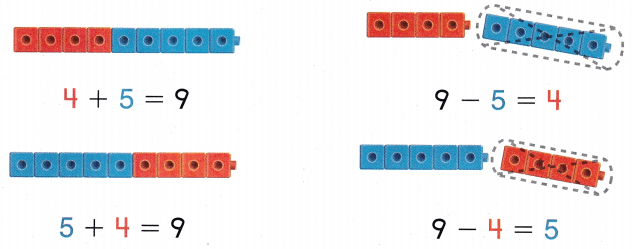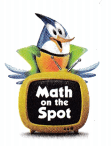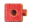# Texas Go Math Grade 1 Lesson 13.1 Answer Key Record Related Facts

Refer to our Texas Go Math Grade 1 Answer Key Pdf to score good marks in the exams. Test yourself by practicing the problems from Texas Go Math Grade 1 Lesson 13.1 Answer Key Record Related Facts.

## Texas Go Math Grade 1 Lesson 13.1 Answer Key Record Related Facts

Explore

Listen to the problem. Model with. Draw. Write the number sentence.
________ + _________ = __________
________ – _________ = __________

FOR THE TEACHER • Read the following problem for the left box. Colin has 7 crackers. He sets 1 more cracker. How many crackers does Colin have now? Then read the following for the right box. Colin has 8 crackers. He gives one to Jacob. How many crackers does Colin have now?

Math Talk
Mathematical Processes

Model and DrawShare and Show

Use. Add or subtract. Complete the related facts.

Question 1.
8 + _________ = 15
7 + 8 = _________

15 – 7 = __________
_________ – _________ = __________
8 + 7 = 15
7 + 8 = 15
15 – 7 = 8
15 – 8 = 7

Question 2.
________ + 9 = 14
9 + 5 = ___________

14 – __________ = 5
_________ – _________ = __________
5 + 9 = 14
9 + 5 = 14
14 – 9 = 5
14 – 5 = 9

Question 3.
7 + _________ = 13
6 + 7 = ____________

13 – 6 = __________
_________ – _________ = __________
7 + 6 = 13
6 + 7 = 13
13 – 6 = 7
13 – 7 = 6

Problem Solving

Use. Add or subtract. Complete the related facts.

Question 4.
________ + 8 = 13
8 + 5 = ____________

13 – __________ = 5
_________ – _________ = __________
5 + 8 = 13
8 + 5 = 13
13 – 8 = 5
13 – 5 = 8

Question 5.
H.O.T. Circle the number sentence that has a mistake. Correct it to complete the related facts.7 + 9 = 16
16 + 9 = 7

9 + 7 = 16
16 – 7 = 9Question 6.
H.O.T. Multi-Step Choose three numbers to make related facts. Choose numbers between 0 and 18. Write your numbers. Write the related facts.Question 7.
Useto count.
Aunt Kay makes 6 instruments. Matt and Karen give her 3 more. Which completes the related facts?
6 + 3 = 9
3 + 6 = 9

9 – 6 = 3
___________
(A) 9 + 3 = 12
(B) 9 – 3 = 6
(C) 6 + 6 = 12
9 – 3 = 6

Question 8.
Analyze Pete has 13 pencils. One day he uses 8 pencils. Another day he uses the rest. Which completes the related facts?
13 – __________ = 8
________ + 8 = 13

13 – 8 = __________
8 + __________ = 13
(A) 6
(B) 8
(C) 5
Given that
The total number of pencils near pete = 13
one day he use pencils = 8
pencils used in another day =13 – 8 = 5
The correct answer is option – C
13 – 5 = 8
5 + 8 = 13
13 – 8 = 5

Question 9.
Texas Test Prep Which completes the related facts?
9 + 8 = 17
8 + 9 = 17

17 – 8 = 9
__________
(A) 9 + 9 = 18
(B) 9 – 6 = 3
(C) 17 – 9 = 8
The correct answer is option – C
9 + 8 = 17
8 + 9 = 17
17 – 8 = 9
17 – 9 = 8

TAKE HOME ACTIVITY • Write an addition fact. Ask your child to write three other related facts.

### Texas Go Math Grade 1 Lesson 13.1 Homework and Practice Answer Key

Add or subtract. Colorto help you. Complete the related facts.Question 1.
_______ + 5 = 11
5 + 6 = ________

11 – _________ = 6
__________ – __________ = __________
6 + 5 = 11
5 + 6 = 11
11 – 5 = 6
11 – 6 = 5

Problem Solving

Circle the number sentence that has a mistake. Correct it to complete the related facts.

Question 2.
6 + 8 = 14
14 – 6 = 8

8 – 6 = 14
14 – 8 = 6Lesson Check

Question 3.
Which completes the related facts?
8 + 4 = 12
4 + 8 = 12

12 – 8 = 4
__________
(A) 12 + 4 = 14
(B) 12 – 4 = 8
(C) 4 + 4 = 8
12 – 4 = 8 is the related fact.
Option B is the correct answer.

Question 4.
Which completes the related facts?
15 – 7 = __________
7 + __________ = 15

15 – ________ = 7
________ + 7 = 15
(A) 8
(B) 9
(C) 7